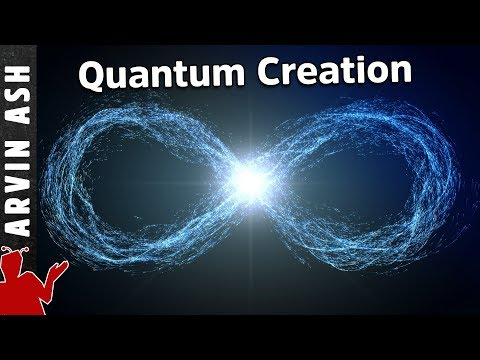Anonymous
Anonymous asked in Society & CultureReligion & Spirituality · 4 weeks ago

# Atheists, if God doesn't exist then how can 3 = 0? How can that be true without the magic of God?

x^2+x+1 = 0 (<=> x+1 = -x^2)

since x can't be 0, we can divide by x

x+1+1/x = 0

substitute x+1 for -x^2

-x^2 + 1/x = 0

1/x = x^2

1 = x^3

x=1

put solution x = 1 it into the original equation x^2+x+1 = 0

1^2+1+1 = 0 or 3 = 0. So, obviously 3 = 0. But how do you explain this fact without God and His powers, atheists?

Update:

Jeremy: You are being DISHONEST. You just picked 5 arbitrarily.

I started with the equation x^2+x+1=0 and moving over the x^2 term gives x+1 = -x^2, so x+1+1/x = -x^2 + 1/x = 0 is true of the equation which I started with for the value of x which solves x^2+x+1 = 0 and is a legal substitution because I was keeping the equation still equal to 0, so it still corresponds to the x that solves the equation, not any random x whatsoever, such as the irrelevant 5, which you just pulled out of your a\$\$

Update 2:

And I wasn't saying that x+1= -x^2 for all values of x. I was clearly working with a special case. I was having the equation equal 0, where that was equivalent to x+1=-x^2

And btw -x^2 for 5 isn't (-5)^2=25, but -(5^2)=-25

Relevance
• This equation isn't even solvable. And your math makes absolutely no sense. Here is your equation graphed. Zoomed in so you can see that the line never shows a possible y value of zero.• well don't

now we know you are stupid as well as unqualified

• I'm pretty sure x=-1. No?

• 0 = matter + gravity

Matter = positive energy

Gravity = negative energy

Thus, according to quantum physics, it is possible (via quantum tunnelling) for the universe to come from empty space (where empty space-time is just quantum fields).

It's explained by some people who are less ignorant than me on the topic here: https://www.youtube.com/watch?v=v8-oocxPwlM•Yoda
Lv 6
4 weeks agoReport

Arvin Ash understands enough to transmit a coherent message---I'm actually going to watch this over a few times before I attempt to explain it to myself

• Your math is nonsense, but you are free to think whatever you like.

• "substitute x+1 for -x^2" NOPE

5+1 = 6

(-5)^2=25

I mean, there are more errors in your math than simply that one. But it boils down to you're substituting values out of the original equation with no justification, solving a second completely new equation, and then saying 3=0. And, this proves your God, how exactly?Edit:  Yes, I picked 5 arbitrarily.  Prefer I do this systematically?  1-100.

1+1=2

(-1)^2=1

2+1=3

(-2)^2=4

3+1=4

(-3)^2=9

4+1=5

(-4)^2=16

5+1=6

(-5)^2=25

6+1=7

(-6)^2=36

7+1=8

(-7)^2=49

8+1=9

(-8)^2=64

9+1=10

(-9)^2=81

10+1=11

(-10)^2=100

11+1=12

(-11)^2=121

12+1=13

(-12)^2=144

13+1=14

(-13)^2=169

14+1=15

(-14)^2=196

15+1=16

(-15)^2=225

16+1=17

(-16)^2=256

17+1=18

(-17)^2=289

18+1=19

(-18)^2=324

19+1=20

(-19)^2=361

20+1=21

(-20)^2=400

21+1=22

(-21)^2=441

22+1=23

(-22)^2=484

23+1=24

(-23)^2=529

24+1=25

(-24)^2=576

25+1=26

(-25)^2=625

26+1=27

(-26)^2=676

27+1=28

(-27)^2=729

28+1=29

(-28)^2=784

29+1=30

(-29)^2=841

30+1=31

(-30)^2=900

31+1=32

(-31)^2=961

32+1=33

(-32)^2=1024

33+1=34

(-33)^2=1089

34+1=35

(-34)^2=1156

35+1=36

(-35)^2=1225

36+1=37

(-36)^2=1296

37+1=38

(-37)^2=1369

38+1=39

(-38)^2=1444

39+1=40

(-39)^2=1521

40+1=41

(-40)^2=1600

41+1=42

(-41)^2=1681

42+1=43

(-42)^2=1764

43+1=44

(-43)^2=1849

44+1=45

(-44)^2=1936

45+1=46

(-45)^2=2025

46+1=47

(-46)^2=2116

47+1=48

(-47)^2=2209

48+1=49

(-48)^2=2304

49+1=50

(-49)^2=2401

50+1=51

(-50)^2=2500

51+1=52

(-51)^2=2601

52+1=53

(-52)^2=2704

53+1=54

(-53)^2=2809

54+1=55

(-54)^2=2916

55+1=56

(-55)^2=3025

56+1=57

(-56)^2=3136

57+1=58

(-57)^2=3249

58+1=59

(-58)^2=3364

59+1=60

(-59)^2=3481

60+1=61

(-60)^2=3600

61+1=62

(-61)^2=3721

62+1=63

(-62)^2=3844

63+1=64

(-63)^2=3969

64+1=65

(-64)^2=4096

65+1=66

(-65)^2=4225

66+1=67

(-66)^2=4356

67+1=68

(-67)^2=4489

68+1=69

(-68)^2=4624

69+1=70

(-69)^2=4761

70+1=71

(-70)^2=4900

71+1=72

(-71)^2=5041

72+1=73

(-72)^2=5184

73+1=74

(-73)^2=5329

74+1=75

(-74)^2=5476

75+1=76

(-75)^2=5625

76+1=77

(-76)^2=5776

77+1=78

(-77)^2=5929

78+1=79

(-78)^2=6084

79+1=80

(-79)^2=6241

80+1=81

(-80)^2=6400

81+1=82

(-81)^2=6561

82+1=83

(-82)^2=6724

83+1=84

(-83)^2=6889

84+1=85

(-84)^2=7056

85+1=86

(-85)^2=7225

86+1=87

(-86)^2=7396

87+1=88

(-87)^2=7569

88+1=89

(-88)^2=7744

89+1=90

(-89)^2=7921

90+1=91

(-90)^2=8100

91+1=92

(-91)^2=8281

92+1=93

(-92)^2=8464

93+1=94

(-93)^2=8649

94+1=95

(-94)^2=8836

95+1=96

(-95)^2=9025

96+1=97

(-96)^2=9216

97+1=98

(-97)^2=9409

98+1=99

(-98)^2=9604

99+1=100

(-99)^2=9801

100+1=101

(-100)^2=10000

Appears your substitution is invalid for all positive integers.  Need me to repeat for negative (Spoilers: Your equation doesn't work there either).

•jon pike
Lv 7
4 weeks agoReport

That's kinda what I thought. If you're solving for x, why do you deem x=1 to begin with?

• You being bad at math is no reason to believe in deities....

• One day trolls will be dragged from their holes And impaled.

~

• Mayans used zero as a base number . How come Mayans know more about the cosmos than flat earth creationist ?

Source(s): Their god makes your god look imaginary
• I once pooped in the forest and 3 months later, I found cantaloupe vine